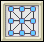Prime Time:  Investigation 1: Factors And Products
 Arnold WorksiteStudent Resources Danicek WorksiteStudent Resources Grimmer WorksiteStudent Resources Homework Help Homework Help Multiple-Choice Skills Practice Multiple-Choice Skills PracticeVocabulary Quiz Insert Video Links Here Pearson Video Tutor Active Math:Core Support Files

# Did You Know?

The Did You Know? feature supports Problems by providing interesting facts related to the context of the Investigation.

Factors

• FunSchool Math Popper Game addition, subtraction, multiplication
• APlusMath-Arithmetic: +-*/
• BBC Bitesize Negative Numbers
• HoodaMath
• Multiplication.Com
• Coolmath
•Math ManThis is a Pac Man type game, but you have to eat the monster that solves the math equation.
•Math Search In this game, you're given an arithmetic problem to solve...  Once you find the answer, you have to find it on the grid of numbers - like in a word search game.
•Number BallsThis is a pretty simple game.  You just click on the balls following the numbers on them from lowest to highest.
•Number Monster This was the first game I ever made.  Really, it's more of a math practice thing than a game.  But, it makes practicing a lot more fun.
•Number Twins  This is like our game, Twins, but we put numbers on the balls.  You have to match up numbers (like in our game Shisen) to make the correct sums.
•Subtraction Blast  I've played this game until my arms were sore.  It's great arithmetic practice while you get to shoot stuff.  Can life get much better than that?
•The Timernator  How fast can you do arithmetic?  Well, with this game, you can find out!  See how many addition, subtraction, multiplication and division problems can you do in a minute?

• National Library of Virtual Manipulatives
•Number Line Bounce – Number line addition and subtraction game.Number Puzzles – Solve puzzles involving arranging numbers on a diagram so that they add up to a given value.
• Cowboy Math Addition and MultiplicationPrime Time
(University Of Michigan)

Concept with Explanation
Selected Homework from ACE

In Prime Time students will explore certain important properties of whole numbers, especially those related to multiplication and division. The investigations in this unit will help students to:

• Understand relationships among factors, multiples, divisors, and products;
• Recognize and use properties of prime and composite numbers, even and odd numbers, and square numbers;
• Use rectangles to represent the factor pairs of numbers;
• Develop strategies for finding factors and multiples, least common multiples, and greatest common factors;
• Recognize and use the fact that every whole number can be written in exactly one way as a product of prime numbers;
• Use factors and multiples to solve problems and to explain some numerical facts of everyday life; and
• Develop a variety of strategies for solving problems building models, making lists and tables, drawing diagrams, and solving simpler problems.
 Vocabulary
 Picture Vocabuary